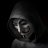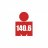# THE WSAC (NEW STRATEGY) for ThinkorSwim

## How do you like the strategy?

• ### hate it,

• Total voters
13
• Poll closed .#### Stocks Rox

##### New member
Hi everyone this is my first time coding on thinkscript so the code really is very easy to understand. WSAC stands for weak trend signal, strong trend signal and confirmed trend signal. Its a very good and reliable swing trading strategy.

BUY SIGNAL CRITERA: Volume EMA for 10 bars is greater then Volume EMA for 35 bars. RSI is less then 45, 5 bars ago. Also price is greater then
a 35 day MA.

SELL SIGNAL CRITERA: Volume EMA for 35 bars is greater then Volume EMA for 10 bars. RSI is greater then 65, 5 bars ago. Also price is less then
a 35 day MA.Here's how it works first if the past ema volume for a more current shorter time period is greater then one of a longer time period, then that's the "W" for a weak bull signal. Then if the RSI is under 45 then that's "S" for the strong trend signal when combined with the "W". Finally if the price crosses the 35 day MA then that's the "AC" and confirmed trend signal (bull). The Bear signal is just vice versa with over bought at 65. Then it will display a green arrow where to buy, and red arrow where to sell.

I like to use it on 5D 5min chart but you can use on anything under 20 days. I tested it in real time on paper money and not in real time. I did only several dozen tests and over 70% of the trades where profitable.

The biggest gain in percent was 220% around and the biggest loss in percent was only 5%!! The average trade has a very small profitable percent at a gain of only 2.5%. Also I only use it to Buy to open, Sell to close but do what you want!

So also i made two indicators that predict signals before it happens but i wont post that till i get feedback from this post. P.S Only use this on stocks that relay on volume, so no ETF's.

Code:
``````#code by YISROEL RIZEL

input R = 14; #hint: its the length of the RSI

input length=35; #hint: its the length of the MA line

input length_longVOLUME=35; #hint: its the volume used to compare the current volume with

input length_currentVOLUME=10;  #hint: its the current volume trend

input BarsAgo=5; #hint: how many bars ago does the program look for a signal from the RSI(-1 for the volume)

input Over_Bought = 65;

input Over_Sold = 45;

def o = open;

def h = high;

def l = low;

def c = close;

def x = BarNumber();

def MidLine = 50;

def NetChgAvg = ExpAverage(c - c, R);

def TotChgAvg = ExpAverage(AbsValue(c - c), R);

def ChgRatio = if TotChgAvg != 0

then NetChgAvg / TotChgAvg

else 0;

def RSI = 50 * (ChgRatio + 1);

def hla=(high+low)/2;

def hlc=(hla+close)/2;

def coa=(close+open)/2;

def coc=(coa+close)/2;

def cccohl=(coc+hlc)/2;

def price=(cccohl+hla)/2;

def source = volume;

def ema1 = expAverage(source,LENGTH_currentVOLUME);

def ema2 = expAverage(source,length_longVOLUME);

def lv = ema1>ema2 ;

def rv= ema1<ema2 ;

def avg = Average(price, length);

def bullma=price crosses above avg;

def bearma=price crosses below avg;

def bullr=RSI from BarsAgo bars ago <Over_Sold;

def bearr=RSI from BarsAgo bars ago >Over_Bought;

AddOrder(OrderType.SELL_TO_CLOSE, bearma and rv and bearr ,open[-1],1,Color.RED, Color.RED,"SELL");

Alert(condition = bearr and rv and bearma, text = "Sell", "alert type" = Alert.BAR, sound = Sound.Chimes);
#hint:The WSAC strategy (weaktrend signal, strong trend signal, and conformed trend signal)S

#END#``````

Last edited:
•murkr, itterichy and RickAns#### BenTen

Staff
VIP
Thank you for sharing, the current P/L from the backtesting data is not reliable because you are using "open" for trade execution rather than "close." To make the result more accurate, I would change it to this:

Code:
``````AddOrder(OrderType.BUY_TO_OPEN, bullma and lv and bullr,close[-1],1,Color.GREEN,Color.GREEN,"BUY");
AddOrder(OrderType.SELL_TO_CLOSE, bearma and rv and bearr ,close[-1],1,Color.RED, Color.RED,"SELL");``````

Edit: My apology, you're already using open[-1]. That should be fine as well.

•Stocks Rox#### Stocks Rox

##### New member
@BenTen OK THX#### BenTen

Staff
VIP
@Stocks Rox Actually, ignore that comment. see my edit.

•Stocks Rox#### Stocks Rox

##### New member
also how if possible can i get email alerts?

A

#### asragov

##### New member
2019 Donor
VIP
I set the code as a strategy and saw losses in 5 day, 5 minute charts for Dow stocks such as AXP, CAT, CSCO, CVX, MRK, etc. Is there some additional filter, different time frame to use, or maybe I am missing something? Thanks very much#### Stocks Rox

##### New member
I set the code as a strategy and saw losses in 5 day, 5 minute charts for Dow stocks such as AXP, CAT, CSCO, CVX, MRK, etc. Is there some additional filter, different time frame to use, or maybe I am missing something? Thanks very much
Your not missing something I looked at all the stocks you wrote with reported losses and all of them hade losses under 4%. Im sure if you look at even
random stocks and follow the strategy you will see a majority of wins. This strategy isn't perfect none are but i relay on it. Also AXP didnt give a sell signal yet so its not a loss, and CVX first lost a dollar per share then gave a buy signal a dollar under the current price but we will see when it calls to sell. Thank you so much for your feedback!#### Stocks Rox

##### New member
Here is a indicator that predicts sell signals
This way you can see the lines stacking up Blue line="W", Pink line="S", and Grey line="AC"
so you dont have to always be looking at your chart and if you missed its very useful.
grey line=3 sell
grey line =2 sometimes sell

Code:
``````#code by YISROEL RIZEL
declare lower;
input R = 14; #hint: its the length of the RSI

input length=35; #hint: its the length of the MA line

input length_longVOLUME=35; #hint: its the volume used to compare the current volume with

input length_currentVOLUME=10;  #hint: its the current volume trend

def BarsAgo=4; #hint: how many bars ago does the program look for a signal from the RSI(-1 for the volume)

input Over_Bought = 65;

input Over_Sold = 45;

def o = open;

def h = high;

def l = low;

def c = close;

def x = BarNumber();

def MidLine = 50;

def NetChgAvg = ExpAverage(c - c, R);

def TotChgAvg = ExpAverage(AbsValue(c - c), R);

def ChgRatio = if TotChgAvg != 0

then NetChgAvg / TotChgAvg

else 0;

def RSI = 50 * (ChgRatio + 1);

def hla=(high+low)/2;

def hlc=(hla+close)/2;

def coa=(close+open)/2;

def coc=(coa+close)/2;

def cccohl=(coc+hlc)/2;

def price=(cccohl+hla)/2;

def source = volume;

def ema1 = expAverage(source,LENGTH_currentVOLUME);

def ema2 = expAverage(source,length_longVOLUME);

def rv= ema1<ema2 ;

def avg = Average(price, length);

def bearma=price crosses below avg;

def bearr=RSI from BarsAgo bars ago >Over_Bought;

plot line1=bearr;

plot line2=bearr+rv;

plot line3=bearr+rv+bearma;
#hint:This Study predicts when then WSAC is going to have a sell signal a bar in avance
#END#``````

•RickAns#### Stocks Rox

##### New member
Here is a indicator that predicts buy signals
This way you can see the lines stacking up Blue line="W", Pink line="S", and Grey line="AC"
so you dont have to always be looking at your chart and if you missed a signal then the price falls or rises and theres a new signal on the indicator the strategy wont show it because it didn't know you missed it so its very useful.
Code:
``````#code by YISROEL RIZEL
declare lower;
input R = 14; #hint: its the length of the RSI

input length=35; #hint: its the length of the MA line

input length_longVOLUME=35; #hint: its the volume used to compare the current volume with

input length_currentVOLUME=10;  #hint: its the current volume trend

def BarsAgo=5; #hint: how many bars ago does the program look for a signal from the RSI(-1 for the volume)

input Over_Bought = 65;

input Over_Sold = 45;

def o = open;

def h = high;

def l = low;

def c = close;

def x = BarNumber();

def MidLine = 50;

def NetChgAvg = ExpAverage(c - c, R);

def TotChgAvg = ExpAverage(AbsValue(c - c), R);

def ChgRatio = if TotChgAvg != 0

then NetChgAvg / TotChgAvg

else 0;

def RSI = 50 * (ChgRatio + 1);

def hla=(high+low)/2;

def hlc=(hla+close)/2;

def coa=(close+open)/2;

def coc=(coa+close)/2;

def cccohl=(coc+hlc)/2;

def price=(cccohl+hla)/2;

def source = volume;

def ema1 = expAverage(source,LENGTH_currentVOLUME);

def ema2 = expAverage(source,length_longVOLUME);

def lv = ema1>ema2 ;

def avg = Average(price, length);

def bullma=price crosses above avg;

def bullr=RSI from BarsAgo bars ago <Over_Sold;

plot line1=bullr;

plot line2=bullr+lv;

plot line3=bullr+lv+bullma;
#hint:this study predicts when the WSAC is going to give a buy signal a bar in advance

#END#``````

•RickAns
F

#### fpapedo

##### New member
VIP
@Stocks Rox Please show a screenshot to explain, would really help me to decide if I like it or not. I like the idea.

•Stocks Rox
N

#### newtoazure

##### New member
Thanks for your input. I've been testing it on the top 10 most active stocks, I'm getting 50% win rate. However, Extremely good returns on Apple, Facebook, Netflix, Tesla, Microsoft. I'm trying to add a buy / sell signal as an alert but getting an error when I do line 1 = 1 .
No such function: BarNumber at 1:1 Expected double. Any idea ?

I'm trying to combine this strategy with a way to find extremely high momentum stocks before they explode. For example PSV on friday. Your strategy triggered a buy signal at 0.55 2 days before. If only I could find it beforehand

Another thing I was trying to do is a scan of buy signals in the morning.

Last edited:
•Stocks Rox
J

#### jay2

##### Member
can you add an alert for the gray line = 3 for the buy? will test this live tomorrow.

•Stocks Rox#### Stocks Rox

##### New member
can you add an alert for the gray line = 3 for the buy? will test this live tomorrow.
Oh thats smart great idea!#### Stocks Rox

##### New member
Thanks for your input. I've been testing it on the top 10 most active stocks, I'm getting 50% win rate. However, Extremely good returns on Apple, Facebook, Netflix, Tesla, Microsoft. I'm trying to add a buy / sell signal as an alert but getting an error when I do line 1 = 1 .
No such function: BarNumber at 1:1 Expected double. Any idea ?

I'm trying to combine this strategy with a way to find extremely high momentum stocks before they explode. For example PSV on friday. Your strategy triggered a buy signal at 0.55 2 days before. If only I could find it beforehand

Another thing I was trying to do is a scan of buy signals in the morning.
Thanks for the feedback Im new to coding on thinkscript and was having trouble getting alerts to my phone or using this in a scan,
When you do make a combination and it works plz share i would love to see that!

Last edited:
C

#### codydog

##### Active member
@Stocks Rox -Thanks for sharing but you may want to consider making your a/c number and how much money in the a/c invisible. Surprised no one else said this.

•Stocks Rox#### Stocks Rox

##### New member
@Stocks Rox -Thanks for sharing but you may want to consider making your a/c number and how much money in the a/c invisible. Surprised no one else said this.
HAHAHA thx 4 ur concern, its my small acount so i wasnt to worried but listened to u.#### Stocks Rox

##### New member
If anyone used this for real plz contact me and tell me how it went I'm very curious! thxMimicking "Power X Strategy" by Markus Heitkoetter Questions 24Create Strategy to open position on 3rd candle above 9 SMA Questions 6